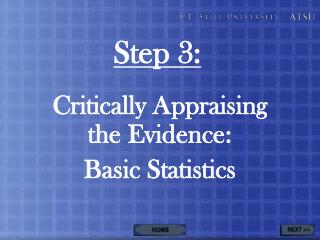DownloadDownload PresentationStep 3:

# Step 3:

Télécharger la présentation## Step 3:

- - - - - - - - - - - - - - - - - - - - - - - - - - - E N D - - - - - - - - - - - - - - - - - - - - - - - - - - -
##### Presentation Transcript

1. Step 3: Critically Appraising the Evidence: Basic Statistics

2. Clinical Statistics Calculator (Excel) Statistics for: General Samples and Populations Means and Medians Normal and Skewed Distributions Variability, Variance, and Standard Deviation Confidence Intervals p-values Generalized 2x2 Clinical Table Practice Exercises Table of Contents

3. If available, find the best evidence in secondary sources where analysis has already occurred. If not pre-assessed, use critical appraisal worksheets to help you through the process. Making It Easier

4. Importance of Critically Appraising the Evidence • Understanding the Limitations of the Author’s Analyses and Interpretations of the Data • Assessing Internal Validity • Assessing External Validity • Identifying Potential Confounding Variables • Simpson’s Paradox

5. Critical Appraisal Basics • View movie as: • QuickTime (.mov) • Flash (.swf) • Double-click on video for full-screen mode.

6. Population vs. Sample • Population • The entire group of all potential patients • Sample • The patients included in the study • Representative of the population • Clinical samples consist of treatment groups • Non-clinical samples consist of control groups

7. Basic Statistical Tools:Mean • Often called arithmetic average or just average • Sum of all of the data points divided by the number of data points

8. Basic Statistical Tools:Median • If there are n data points lined up in numerical order the median is the one in the exact middle or the average of the 2 middle points if there is an even number of data points. In other words it is the nth/2 data point if n is odd and the ((n-1)th/2 + (n+1)th/2)/2 data point if n is even.

9. Median:In Layman’s Terms • Line up all of the data points in increasing order. • The one in the middle is the median. • If there is no clear single mid-point (i.e. there is an even number of data points), the median is half-way between the two middle points. • So if 0, 1, 2, 4 were our data set, 1.5 would be the median.

10. Normal vs. Skewed • Normal • Symmetric, bell-shaped distribution where the frequency of data within an interval is greater the nearer it is to the mean • Skewed • Asymmetric distribution • More data to one side of the mean than the other

11. Mean vs. Median • Additional outliers • usually increase/decrease mean more than median • Skewed data • Mean shifted toward the tail (i.e. the side where the data points are more spread out) • Median unaffected by distribution/shape • Always the middle value regardless of where the other points lie

12. When is the distinction between mean and a median important? • Mean (i.e. Arithmetic Average) • Used when data is approximately normally distributed • Median • Sometimes used with skewed data due to its robustness • Preferred on Likert scales (survey data) since values are ranked but their differences are not clearly quantifiable. • After all, what number does “agree” minus “disagree” equal? Regardless of how one quantifies these differences, the median remains the same, but the mean depends on the scale.

13. If mean and median greatly differ • either: • the data is not normal and is skewed one way or another • there is at least one outlier with a lot of leverage

14. Basic Statistical Tools:Measures of Variability • Variability / Dispersion • How data points are distributed • Variance • Sum of the squares of the differences between each data point and the sample mean, divided by the total number of data points • Standard Deviation • Square root of the variance

15. Confidence Intervals • Range in which one would expect the values of the outcome variables to fall into when replicating the experiment given a quantifiable probability of error.

16. p-value • Probability of obtaining a result as extreme as the observed one if the data were a result of chance. • Small p-values mean the result could not likely be the result of chance. • Often researches use p-values such as 0.10, 0.05, 0.01, and smaller.

17. Generalized 2x2 Clinical Table

18. Try it on your own. • Critical Appraisal Practice Exercises • From CEBM

19. Links to Other Websitesand Hands-On Activities • EBM Glossary • From CEBM • Critical Appraisal Practice Exercises • From CEBM

20. Congratulations!You have successfully completed Step 3: Basic Statistics.The End